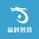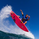# Spiky Iguana Multi RSI Bands (Reverse RSI for the Price) by RRB

צפיות ‎295‎
295
Spiky Iguana Multi RSI Bands by RagingRocketBull 2018
Version 1.0

This indicator shows multiple RSI Bands with prices corresponding to specified overbought/oversold RSI levels.
It is used to extrapolate the exact price levels currently matching a given set of RSI levels based on prior price/RSI levels action.
You can think of it as a reverse RSI where RSI levels are moving dynamically around the price instead of price bouncing between straight lines.

Features:
- 6+6 = 12 customizable Overbought(R)/Oversold(S) RSI Levels + 6 Mean(M) lines
- Multicolor levels/fill ranges
- Show/Hide specific S/R/M levels and fill ranges

1. uses plot*, fill and is based on RSIBANDS_LB

Good Luck! Feel free to explore and learn from the codeVery beautiful code.
x = (len - 1) * ( adc * level / (100-level) - auc)
I don't quite understand this sentence. What logic is it based on? Can you explain it? Thx.
השבthis indicator is based on RSIBANDS_LB. Perhaps, LB himself can better explain how reverse RSI is calculated. I modified his code to add more levels.

RSI Levels are specified in percentages , so some sort of normalization is needed for x - that's what that line does.
auc = AvgU = average of all up moves in the last ep price bars
adc = AvgD = average of all down moves in the last ep price bars

This is how RSI is calculated (script uses the same formula):